2. Theory
2.1 Contour Dynamics
2.2 Equations of Cotour Dynamics
2.3 Theory of an Elliptical Vortex
2.4 Finite-Time Lyapunov Analysis on Fluid Deformation

### 2.2 Equations of Cotour Dynamics

Basics of contour dynamics are summarized following Dritschel(1989).

The streamfunction Y( x,y,t ) is related to the vorticity Q( x,y,t ) through Poisson's equation,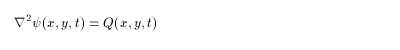(2.3)
General solution can be obtained using the Green function G(r):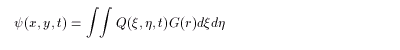(2.4)
where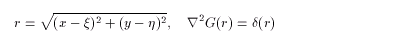(2.5)

As for the two-dimensional infinite plane, G(r) = ( log r )/ 2p :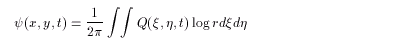(2.6)
The velocity (u,v) is given by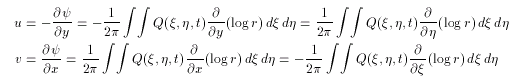(2.7)

By integrating (2.7) partially by h,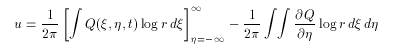(2.8)
The h integral of the second part of the right-hand side is equal to the vorticity jump q( j ) , since Q/¶h≠0 only on the contour. Letting q( j ) be the vorticity jump crossing inward, and taking the line integral anti-clockwise, we obtain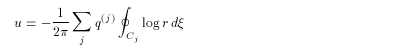(2.9)
Calculating v in the same way yields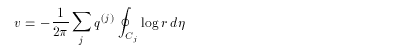(2.10)

These two equations are bound up to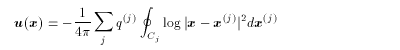(2.11)
which means that the velocity at an arbitrary point can be calculated from the contour position { x( j ) } . Since the velocity on the contour can be calculated. Time evolution of the contour can be computed by advecting the contour using that velocity.

<<index <previous next>